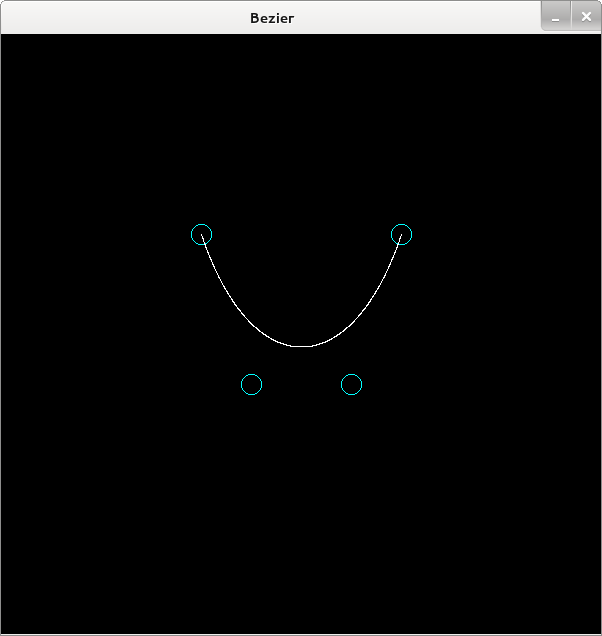# Bezier曲線

```(defstruct point x y)

(defun point (x y)
(make-point :x x :y y))

(defmethod point-diff ((p1 point) (p2 point))
(sqrt (+ (expt (- (point-x p1) (point-x p2)) 2)
(expt (- (point-y p1) (point-y p2)) 2))))

(defstruct line p1 p2)

(defmethod line ((p1 point) (p2 point))
(make-line :p1 p1 :p2 p2))

;;division point of line i:1-i
(defmethod div-point ((l line) i)
(let ((x-diff (- (point-x (line-p2 l)) (point-x (line-p1 l))))
(y-diff (- (point-y (line-p2 l)) (point-y (line-p1 l)))))
(make-point
:x (+ (point-x (line-p1 l)) (* x-diff (/ i 1)))
:y (+ (point-y (line-p1 l)) (* y-diff (/ i 1))))))

(defun inter (list fn)
(mapcar fn
list
(cdr list)))

(defun %bezier (time &rest control-points)
(mapcar (lambda (l)
(div-point l time))
(inter control-points #'line)))

(defun %%bezier (time &rest control-points)
(loop for points = (apply #'%bezier `(,time ,@control-points)) then (apply #'%bezier `(,time ,@points))
until (= 1 (length points))
finally (return (car points))))

(defun bezier (&rest control-points)
(loop for time from 0.0 upto 1.0 by 0.001
collect
(apply #'%%bezier `(,time ,@control-points))))

(defun bezier-viewer (&rest control-points)
(let ((points (apply #'bezier control-points)))
(sdl:with-init ()
(sdl:window 600 600 :title-caption "Bezier")
(sdl:clear-display sdl:*black*)
;;Draw ControlPoint
(loop for cpoint in control-points
do
(sdl:draw-circle-* (round (point-x cpoint)) (round (point-y cpoint)) 10 :color sdl:*cyan*))
;;Draw Bezier Curve
(loop for point in points
do
(sdl:draw-pixel-* (round (point-x point)) (round (point-y point)) :color sdl:*white*))
(sdl:update-display)
(sdl:with-events ()
(:quit-event () t)
(:idle () (sdl:update-display))))))
```

こんなかんじ

```CL-USER(65): (bezier-viewer (point 200 200) (point 250 350) (point 350 350) (point 400 200))
```